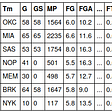# How can I change a cell array with timepoints in to a continous

How can I change a cell array with timepoints in to a continous binary matrix? (for Neural Network Toolbox)

I have a 15x1 cell array, in which each cell is another 50x2 cell. The data shows spike-event onsets in seconds for 50 neurons (each row is a neuron). The content looks like this:

`(XX{1,1}) =     {[  3.4078]}    {[  3.7273]}    {0×0 double}    {0×0 double}    {0×0 double}    {0×0 double}    {[  3.4684]}    {0×0 double}      ...`

If this data is used for network training, this is the error that I get:

`Error using trainNetwork (line 140)Invalid training data. Predictors must be a cell array of sequences. The data dimension of all sequences must be the same.Caused by:  Error using nnet.internal.cnn.util.TrainNetworkDataValidator/assertValidSequenceInput (line 269)  Invalid training data. Predictors must be a cell array of sequences. The data dimension of all sequences must be the same.`

In order to use this as an input for a LSTM-Network in the Neural Network Toolbox these timepoints needs to be part of a continous spectrum. My idea was to convert this discrete datapoints in to large binary matrix (50x10000) inwhich the columns represent the time, therefore 10 seconds = 10000 columns. At each timepoint a spike occured (e.g. 3.4078) a 1 should be put for the corresponding neuron. I tried indexing the cell array with a time-vector ( t = (0:0.001:10) ) but it didn’t work. Can anyone help transforming the data? Thank you.

Matlabsolutions.com provide latest MatLab Homework Help,MatLab Assignment Help for students, engineers and researchers in Multiple Branches like ECE, EEE, CSE, Mechanical, Civil with 100% output.Matlab Code for B.E, B.Tech,M.E,M.Tech, Ph.D. Scholars with 100% privacy guaranteed. Get MATLAB projects with source code for your learning and research.

If I am understanding you correctly, you would like to change each 50x2 cell array into a 50x10000 matrix of mainly zeros, with a value of 1 at indices that correspond to the times in the original 50x2 array. You can do this by making a new 15x1 cell array in which each cell is a 50x10000 matrix of zeros. Then, use the times in the original array to index into the matrices of zeros to set the corresponding entry to 1. This would look something like:

`% XX = original cell arrayseqNum = 15;neuronNum = 50;% create new cell arrayXX2 = cell([seqNum 1]);for i = 1:seqNum    XX2{i} = zeros(neuronNum, 10000);    currSeq = XX{i};    for j = 1:neuronNum`

https://www.matlabsolutions.com/resources/how-can-i-change-a-cell-array-with-timepoints-in-to-a-continous-.php

--

--

--

## More from Technical Source

Simple! That is me, a simple person. I am passionate about knowledge and reading. That’s why I have decided to write and share a bit of my life and thoughts to.

Love podcasts or audiobooks? Learn on the go with our new app.

## Understanding Optical Flow & RAFT## Assessing similarity using K-means Clustering## Build a fully production ready machine learning app with Python Django, React and Docker## Multimodal deep learning models for early detection of Alzheimer’s disease stage## Build Recommendation Systems with PyTorch Geometric and ArangoDB## Text Understanding with Convolutional Neural Network with Hands-on Coding## Ensemble Techniques## Embeddings with Word2Vec in non-NLP Contexts — Details## Technical Source

Simple! That is me, a simple person. I am passionate about knowledge and reading. That’s why I have decided to write and share a bit of my life and thoughts to.

## What’s the most efficient way to isolate a connected## End to End Machine Learning with H2O.ai## FastAI Bag of Tricks — Hand Sign Classification